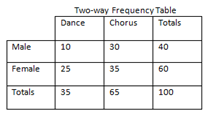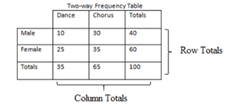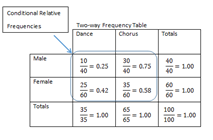# Access Point #: MAFS.912.S-CP.1.AP.3a

Using a two-way table, find the conditional probability of A given B.
Clarifications:

#### Essential Understandings

Concrete:

• Create a two-way table using objects/manipulatives.• List the possible combinations to create a two-way table (e.g., using one male figurine, twelve matchbox cars, and eight toy SUV’s to model the probability of the type of car given the customer is a male).
Representation:
• Understand that a two-way table looks at sub groups within the whole group. For example, looking at how boys scored on a test verses how girls scored on the same test.
• Use this two-way table to determine specific probabilities. E.g., the probability of the owner being female given a sports car is 45 out of 84 or 0.54.• Use this two-way table to determine specific probabilities. E.g., the probability of choosing a sports car given they are a male in the table below is 39 out of 60 or 0.65.General Information
Number: MAFS.912.S-CP.1.AP.3a
Category: Access Points
Cluster: Understand independence and conditional probability and use them to interpret data. (Algebra 2 - Additional Cluster)

Clusters should not be sorted from Major to Supporting and then taught in that order. To do so would strip the coherence of the mathematical ideas and miss the opportunity to enhance the major work of the grade with the supporting clusters.

## Related Standards

This access point is an alternate version of the following benchmark(s).

## Related Courses

This access point is part of these courses.
1200330: Algebra 2
1200340: Algebra 2 Honors
1210300: Probability and Statistics Honors
1200335: Algebra 2 for Credit Recovery
7912095: Access Algebra 2

## Related Resources

Vetted resources educators can use to teach the concepts and skills in this access point.

## Student Resources

Vetted resources students can use to learn the concepts and skills in this access point.

## Parent Resources

Vetted resources caregivers can use to help students learn the concepts and skills in this access point.